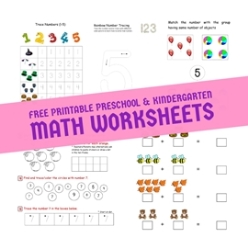Looking for Free Printable Kindergarten Math Worksheets or Preschool Math Worksheets for your child? From number recognition to counting, number formation, tracing, ordering, more or less concept, basic addition and subtraction we have free math worksheets for all the learning requirements of your child. Do check our hundreds of free printable preschool and kindergarten math worksheets here.

Ten Frame is a simple yet powerful mathematical tool to learn counting, number sense, addition, subtraction, even/odd, number relationships and many other concepts to a child. It also improves subitizing skills. Here we provide a variety of ten frame worksheets to help learn various math concepts.

Ten frames is a great mathematical tool for teaching counting, addition, subtraction, number sense to children. Here we are offering you some blank ten frame and double ten frame templates. These ten frame templates can be used in your own way. You can color, place counters/manipulatives or paste stickers in cells of ten-frames to represent any number. They also help understand the decimal place value system in higher grades (e.g., 14 can be represented as 10 + 4).

If you are looking for math activities for preschoolers/kindergartener, you are at the right place. We have a collection of free printables for engaging and fun math activities. Your child is going to love learning numbers with these interesting activities.

Check out these Number Recognition Activity Sheets which are specifically designed for beginner/pre-k level.

Check out these free printable Addition and Subtraction Word Problems worksheets to help your child understand the application of addition/subtraction in daily life. Step-by-step examples to solve the addition/subtraction story problems help beginners grasp the concept.

The big bold numbers are traced several times using crayons or color pencils to understand the number formation.

Check these worksheets to introduce your child to Number Subtraction (1-10) using various techniques( Subtract with pictures/objects/tally marks to cross out, using number line).

Looking for worksheets to teach addition to your child? Our free printable addition worksheets would teach them Number Addition (1-10) using various techniques (Addition With Pictures/Objects, Addition Using Number Line and Tally Marks) and establish the concept that Addition is Commutative.

Check out these worksheets to help your child learn number counting (1-10) in different ways using our free printable worksheets like count and write numbers, count the objects of different types, count and color the correct number of objects etc.

Want to improve your child's counting skills. Check out our collection of free printable counting and number matching worksheets - Count the objects and match with the number, Match the groups with same number of objects, Match number with groups containing same number of objects.

These free printable worksheets for comparing numbers/quantities teach the concept of more or less, greater than less than to your child. Gradually children can be introduced to comparison signs like greater than, less than and equals to (>, < and =).

Learn ordering numbers by using our various free printable number order worksheets on what comes before and after a number as well as fill the missing numbers.

These number tracing worksheets are designed to help child learn to trace and write numbers (1-10) with understanding the counting.